# Consider a 50 mL solution of .55M CaCl2 (aq). a solid piece of NaCl (s) with...

Consider a 50 mL solution of .55M CaCl2 (aq). a solid piece of NaCl (s) with a volumw of 9.5 cm^3 is added to the aqueous calcium chloride solution. Calculate the chloride ion concentration in the mixture after the solid completely dissolves.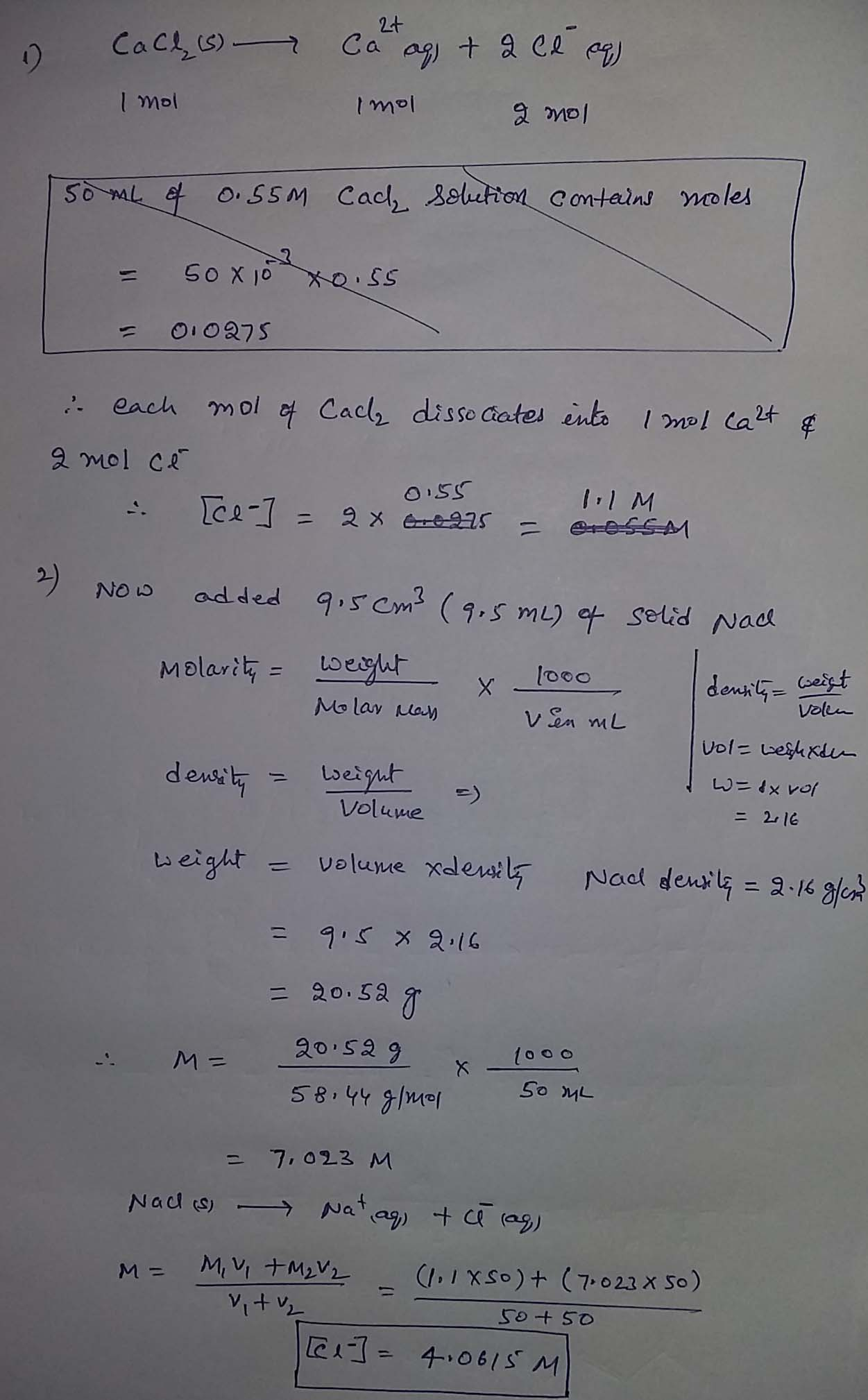#### Earn Coin

Coins can be redeemed for fabulous gifts.

Similar Homework Help Questions
• ### Name: 1.00g NaCl Volume: 100.34 mL Species (aq) a) The solution labeled 'Solution 1' contains 2.00...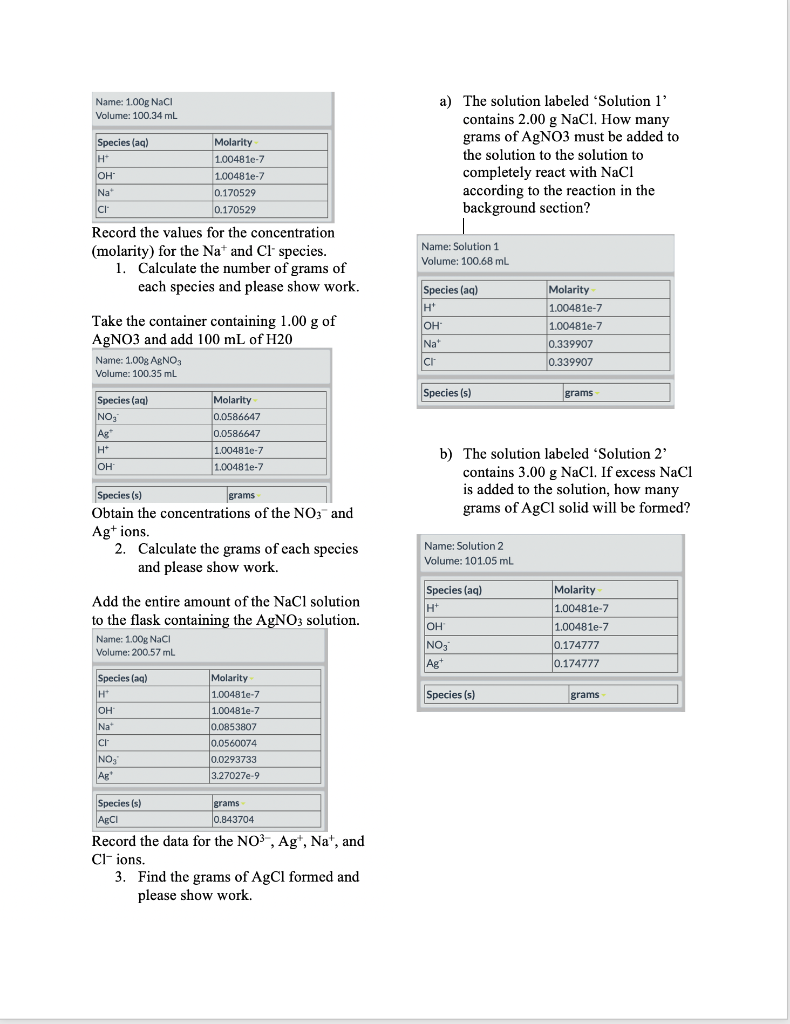Name: 1.00g NaCl Volume: 100.34 mL Species (aq) a) The solution labeled 'Solution 1' contains 2.00 g NaCl. How many grams of AgNO3 must be added to the solution to the solution to completely react with NaCl according to the reaction in the background section? Molarity 1.00481e-7 1.00481e-7 0.170529 0.170529 OH Na CI Record the values for the concentration (molarity) for the Nat and Cl' species. 1. Calculate the number of grams of each species and please show work. Name:...

• ### (7. Consider the reaction as follows: Na2SO4(aq) + BaCl2(aq) → BaSO4(s) + 2 NaCl(aq) A 1.00-mol sample of sodium...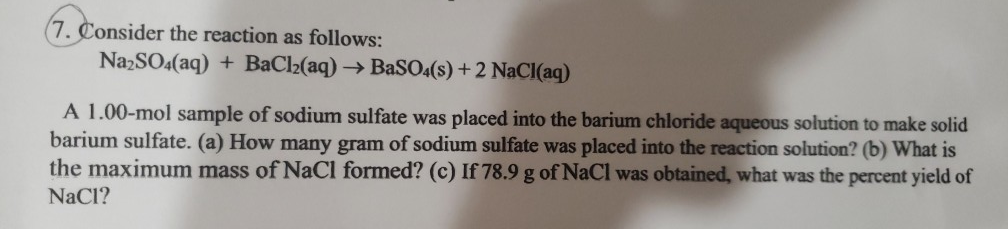(7. Consider the reaction as follows: Na2SO4(aq) + BaCl2(aq) → BaSO4(s) + 2 NaCl(aq) A 1.00-mol sample of sodium sulfate was placed into the barium chloride aqueous solution to make solid barium sulfate. (a) How many gram of sodium sulfate was placed into the reaction solution? (b) What is the maximum mass of NaCl formed? (c) If 78.9 g of NaCl was obtained, what was the percent yield of NaCl? 3. How many liters of 0.186 M of NaOH (aq)...

• ### Suppose a 12.42g mixture of NaCl and CaCl2 is dissolved in water. To this solution, an...

Suppose a 12.42g mixture of NaCl and CaCl2 is dissolved in water. To this solution, an excess of AgNO3 solution is added resulting in the formation of 31.12g of the precipitate. Calculate the % by mass of NaCl and CaCl2 in the original mixture. Show all work.

• ### 6. A 50.0 mL sample of 0.528 M NHaNO, is diluted with water to a total...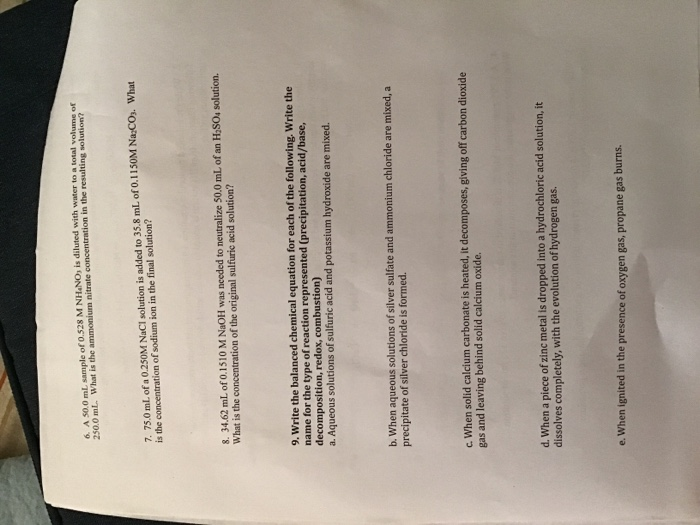6. A 50.0 mL sample of 0.528 M NHaNO, is diluted with water to a total volume of 250.0 mL What is the ammonium nitrate concentration in the resulting solution? 7. 75.0 ml. of a 0.250M NaCI solution is added to 35.8 ml. of 0.1150M NaCOs. What is the concentration of sodium ion in the final solution? 8, 34.62 mL. of 0.1510 M NaOH was needed to neutralize 50.0 mL of an H2SO4 solution. What is the concentration of the...

• ### Suppose a 12.42g mixture of NaCl and CaCl2 is dissolved in water. To this solution, an excess of AgNO3 solution is added...

Suppose a 12.42g mixture of NaCl and CaCl2 is dissolved in water. To this solution, an excess of AgNO3 solution is added resulting in the formation of 31.12g of the precipitate. Calculate the % by mass of NaCl and CaCl2 in the original mixture. Show all work.

• ### 1. Consider the following equation: Ca(OH)2(s) + 2HCl(aq) + CaCl2(aq) + 2 H20(1).Solve the following questions...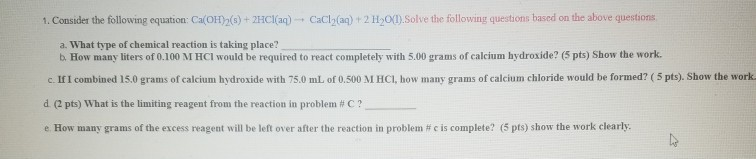1. Consider the following equation: Ca(OH)2(s) + 2HCl(aq) + CaCl2(aq) + 2 H20(1).Solve the following questions based on the above questions, a. What type of chemical reaction is taking place? b. How many liters of 0.100 M HCI would be required to react completely with 5.00 grams of calcium hydroxide? (5 pts) Show the work. c. If I combined 15.0 grams of calcium hydroxide with 75,0 mL of 0.500 M HCI, how many grams of calcium chloride would be formed?...

• ### Silver ions can be precipitated from aqueous solutions by the addition of aqueous chloride: Ag+(aq)+Cl−(aq)→AgCl(s) Silver...

Silver ions can be precipitated from aqueous solutions by the addition of aqueous chloride: Ag+(aq)+Cl−(aq)→AgCl(s) Silver chloride is virtually insoluble in water so that the reaction appears to go to completion. How many grams of solid NaCl must be added to 25.0 mL of 0.149 M AgNO3 solution to completely precipitate the silver?

• ### A solution is prepared by mixing 100 mL of 2M NaCl, 100 mL of 1M CaCl2...

A solution is prepared by mixing 100 mL of 2M NaCl, 100 mL of 1M CaCl2 and 650 mL of water. What is the molar ratio of Ca:Cl:Na in the final solution? please show full solution, (Indicate your answers to two digits after the decimal)

• ### If we have 50 mL of a 1.0M sodium hydroxide solution and 50 mL of a...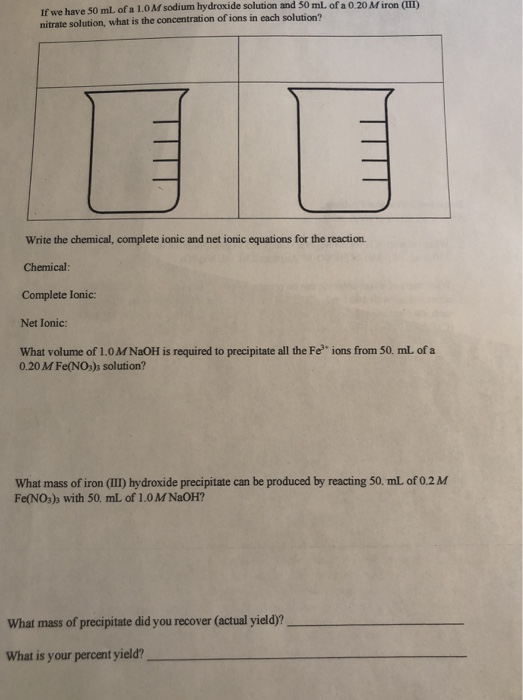If we have 50 mL of a 1.0M sodium hydroxide solution and 50 mL of a 0.20 M iron (III) nitrate solution, what is the concentration of ions in each solution? Write the chemical, complete ionic and net ionic equations for the reaction. Chemical: Complete lonic: Net Ionic: What volume of 1.0M NaOH is required to precipitate all the Fe ions from 50. mL of a 0.20 M Fe(NO) solution? What mass of iron (II) hydroxide precipitate can be produced...

• ### Ca(OH)2 (aq) + 2HCl (aq) CaCl2 (aq) + H2​O (l) An aqueous solution of Ca(OH)2with a...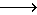Ca(OH)2 (aq) + 2HCl (aq) CaCl2 (aq) + H2​O (l) An aqueous solution of Ca(OH)2with a concentration of 0.161 M was used to titrate 25.00 mL of aqueous HCl. 18.63 mL of the Ca(OH)2was required to reach the endpoint of the titration. An aqueous solution of Ca(OH)2with a concentration of 0.161 M was used to titrate 25.00 mL of aqueous HCl. 18.63 mL of the Ca(OH)2was required to reach the endpoint of the titration. A) How many moles of base...